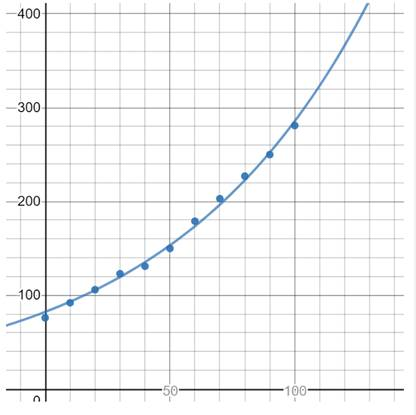# To model: The population of US with data from 1900 − 2000 and then estimate the in 1925 and predict the population in the year 2010 and 2020 .### Single Variable Calculus: Concepts...

4th Edition
James Stewart
Publisher: Cengage Learning
ISBN: 9781337687805### Single Variable Calculus: Concepts...

4th Edition
James Stewart
Publisher: Cengage Learning
ISBN: 9781337687805

#### Solutions

Chapter 1.5, Problem 34E
To determine

## To model:The population of US with data from 1900−2000 and then estimate the in 1925 and predict the population in the year 2010 and 2020 .

Expert Solution

### Explanation of Solution

The model of the population for the year 1900 to 2000 is shown in graph below.Figure (1)

The best fitting exponential model for the data is,

y=82.2584(1.13258)x ....... (1)

Substitute 25 for x in equation (1).

y=82.2584(1.13258)25112

Substitute 110 for x in equation (1).

y=82.2584(1.13258)110324

Substitute 120 for x in equation (1).

y=82.2584(1.13258)120367

Therefore, the exponential model is y=82.2584(1.13258)x , the estimated population in year 1925 is 112million , predicted population in 2010 is 324million and in 2020 is 367million .

### Have a homework question?

Subscribe to bartleby learn! Ask subject matter experts 30 homework questions each month. Plus, you’ll have access to millions of step-by-step textbook answers!